Next: Interpretation of conditional probability Up: Appendix on probability and Previous: Unifying role of subjective   Contents

# Frequentists and combinatorial evaluation of probability

In the previous section it was was said that frequentists should abstain from assessing probabilities if a long-run experiment has not been carried out. But frequentists do, using a sophisticated reasoning, of which perhaps not everyone is aware. I think that the best way to illustrate this reasoning is with an example of an authoritative exponent, Polya, who adheres to von Mises' views.
A bag contains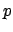balls of various colors among which there are exactly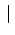white balls. We use this simple apparatus to produce a random mass phenomenon. We draw a ball, we look at its color and we write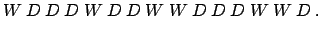if the ball is white, but we writeif it is of a different color. We put back the ball just drawn into the bag, we shuffle the balls in the bag, then we draw again one and note the color of this second ball,or. In proceeding so, we obtain a random sequence (...):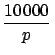What is the long range relative frequency of the white balls?

Let us assume that the balls are homogeneous and exactly spherical, made of the same material and having the same radius. Their surfaces are equally smooth, and their different coloration influences only negligibly their mechanical behavior, if it has any influence at all. The person who draws the balls is blindfolded or prevented in some other manner from seeing the balls. The position of the balls in the bag varies from one drawing to the other, is unpredictable, beyond our control. Yet the permanent circumstances are well under control: the balls are all the same shape, size, and weight; they are indistinguishable by the person who draws them.

Under such circumstances we see no reason why one ball should be preferred to another and we naturally expect that, in the long run, each ball will be drawn approximately equally often. Let us say that we have the patience to make 10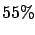000 drawings. Then we should expect that each of theballs will appear about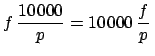times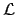There arewhite balls. Therefore, in 10000 drawings, we expect to get white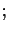times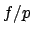this is the expected frequency of the white balls. To obtain the relative frequency, we have to divide by the number of observations, or drawings, that is, 10000. And so we are led to the statement: the long range relative frequency, or probability, of the white balls is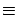.

The lettersandare chosen to conform to the traditional mode of expression. As we have to draw one of theballs, we have to choose one ofpossible cases. We have good reasons (equal condition of theballs) not to prefer any of thesepossible cases to any other. If we wish that a white ball should be drawn (for example, if we are betting on white), thewhite balls appear to us as favourable cases. Hence we can describe the probabilityas the ratio of the number of favourable cases to the number of possible cases.''
The approach sketched in the above example is based on the refusal of calling probability (the intuitive concept of it) by its name. The term probability' is used instead for long-range relative frequency'. Nevertheless, the value of probability is not evaluated from the information about past frequency, but from the hypothetical long-range relative frequency, based on: a) plausible (and subjective!) reasoning on equiprobability (although not stated with this term) of the possible outcomes; b) the expectation (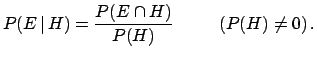belief) that the relative frequency will be equal to the fraction of white balls in the bag.8.5 The overall result is to confuse the matter, without any philosophical or practical advantages (compare the twisted reasoning of the above example with Hume's lucid exposure of the concept of probability and its evaluation by symmetry arguments, reported in Section).Next: Interpretation of conditional probability Up: Appendix on probability and Previous: Unifying role of subjective   Contents
Giulio D'Agostini 2003-05-15